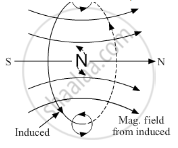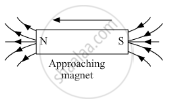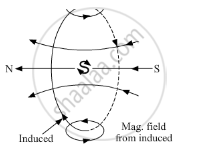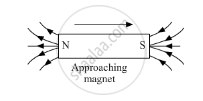# State Lenz'S Law. Illustrate, by Giving an Example, How this Law Helps in Predicting the Direction of the Current in a Loop in the Presence of a Changing Magnetic Flux. - Physics

State Lenz's law. Illustrate, by giving an example, how this law helps in predicting the direction of the current in a loop in the presence of a changing magnetic flux.

#### Solution

(i)According to Lenz's law, the polarity of emf induced in the coil always opposes the change in the magnetic flux responsible for production of induced emf.

(ii) Lenz's law is used to determine the direction of current induced in the closed circuit. Let us consider an example to illustrate it. When a north pole of a bar magnet is brought closer to a coil, as shown in figure, the amount of magnetic flux linked with the coil increases. So, the current induced in the coil is directed such that it opposes this change in magnetic flux responsible for production of induced current, which is possible only when the current induced in the coil is in anticlockwise direction of the observer on the side of the magnet.
Similarly, the direction of induced current can be found when north pole of bar magnet is moved away from the coil. As the magnetic flux in this case decreases, the induced current is in the clockwise direction.\Concept: Lenz’S Law and Conservation of Energy
Is there an error in this question or solution?